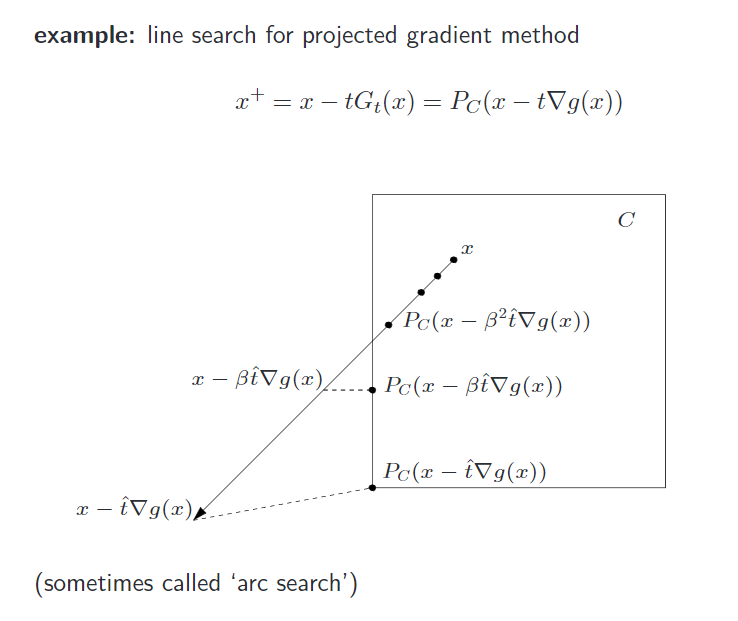# 近端梯度下降——Proximal Method

## L1 范数问题求解

Posted by Roach on August 3, 2016

#### 性质

性质 (condition for global minimizer). 点 $x_0$ 是凸函数 $f$ 的一个全局最小值点，当且仅当 $0 \in \partial f(x_0)$.

## proximity operator

• $\varphi(x)=0: \mbox{prox}_{\gamma\varphi}(x)=x$
• $\varphi(x)=I_C(x) \; \mbox{(indicator function of C): } \mbox{prox}_{\varphi} \mbox{ is projection on C }$
• $\varphi(x)= \mid\mid x\mid\mid_1 : \mbox{prox}_{\varphi} \mbox{is shrinkage (soft threshold) operation}$

$I_C(x)$ 表示如果 $x$ 在集合中则函数值为 $0$，否则为正无穷；$\varphi(x)= \mid\mid x\mid\mid_1$的情况下一节进行进一步讨论。

#### soft threshold

$R(x)=\varphi(x)= \mid\mid x\mid\mid_1$，根据性质：若 $x_0$ 是凸函数 $f(x)$ 的极值点，那么 $x_0 \in \partial f(x)$，得，

$\because u\in x-\gamma\partial\mid u\mid \And\ \partial \mid 0\mid=[-1,1]\therefore u=0 \in x-[-\gamma, \gamma] \Rightarrow 0 \in x+[-\gamma, \gamma]$，也就是说0在区间$x+[-\gamma, \gamma]$内，则$x \in [-\gamma, \gamma]$。

soft thresholding operator 为 $S_{\gamma }(x)= \mbox{prox} _{\gamma \mid\mid \cdot \mid\mid_1}(x)$

#### 证明

###### 凸函数下界

affine lower bound from convexity 凸函数的仿射下界：

## 线性搜索#### 符号化

start at some, $t:= \hat t$,repeat,$t:=\beta t$ (with 0<β<1), until:

## 小结

• 二阶泰勒近似，使用了 利普希茨 条件，但是这一部分尚没有搞清楚
• 有一个参考文献中，设置了类似于 Proximal Gradient Method 的步长，个人偏向不用设置步长

## 附加

$\mbox{l}1$norm 稀疏化的一个解释（非明了的图形），待解决问题即 $LASSO$ 部分所列问题，若在 $0$ 处， $F’(x)<\gamma \mid_{x=0}$，则 $0 \in \partial \left(F(x)+\gamma \mid\mid x \mid\mid_1 \right) \mid_{x=0}$，则得稀疏解

$\mbox{l}2$norm 则更倾向于平滑，待解决问题：